Note

This page is a reference documentation. It only explains the class signature, and not how to use it. Please refer to the user guide for the big picture.

# nilearn.decoding.SpaceNetRegressor#

class nilearn.decoding.SpaceNetRegressor(penalty='graph-net', l1_ratios=0.5, alphas=None, n_alphas=10, mask=None, target_affine=None, target_shape=None, low_pass=None, high_pass=None, t_r=None, max_iter=200, tol=0.0001, memory=Memory(location=None), memory_level=1, standardize=True, verbose=1, n_jobs=1, eps=0.001, cv=8, fit_intercept=True, screening_percentile=20.0, debias=False)[source]#

Regression learners with sparsity and spatial priors.

SpaceNetRegressor implements Graph-Net and TV-L1 priors / penalties for regression problems. Thus, the penalty is a sum an L1 term and a spatial term. The aim of such a hybrid prior is to obtain weights maps which are structured (due to the spatial prior) and sparse (enforced by L1 norm).

Parameters:
penaltystring, optional (default ‘graph-net’)

Penalty to used in the model. Can be ‘graph-net’ or ‘tv-l1’.

l1_ratiosfloat or list of floats in the interval [0, 1]; optional (default .5)

Constant that mixes L1 and spatial prior terms in penalization. l1_ratios == 1 corresponds to pure LASSO. The larger the value of this parameter, the sparser the estimated weights map. If list is provided, then the best value will be selected by cross-validation.

alphasfloat or list of floats, optional (default None)

Choices for the constant that scales the overall regularization term. This parameter is mutually exclusive with the n_alphas parameter. If None or list of floats is provided, then the best value will be selected by cross-validation.

n_alphasint, optional (default 10).

Generate this number of alphas per regularization path. This parameter is mutually exclusive with the alphas parameter.

epsfloat, optional (default 1e-3)

Length of the path. For example, eps=1e-3 means that alpha_min / alpha_max = 1e-3

Mask to be used on data. If an instance of masker is passed, then its mask will be used. If no mask is it will be computed automatically by a MultiNiftiMasker with default parameters.

target_affinenumpy.ndarray, default=None

If specified, the image is resampled corresponding to this new affine. target_affine can be a 3x3 or a 4x4 matrix.

target_shapetuple or list, default=None

If specified, the image will be resized to match this new shape. len(target_shape) must be equal to 3.

Note

If target_shape is specified, a target_affine of shape (4, 4) must also be given.

low_passfloat or None, default=None

Low cutoff frequency in Hertz. If specified, signals above this frequency will be filtered out. If None, no low-pass filtering will be performed.

high_passfloat, default=None

High cutoff frequency in Hertz. If specified, signals below this frequency will be filtered out.

t_rfloat or None, default=None

Repetition time, in seconds (sampling period). Set to None if not provided.

screening_percentilefloat in the interval [0, 100]; Optional (default 20)

Percentile value for ANOVA univariate feature selection. A value of 100 means ‘keep all features’. This percentile is expressed w.r.t the volume of a standard (MNI152) brain, and so is corrected at runtime to correspond to the volume of the user-supplied mask (which is typically smaller).

standardizebool, optional (default True):

If set, then we’ll center the data (X, y) have mean zero along axis 0. This is here because nearly all linear models will want their data to be centered.

fit_interceptbool, optional (default True)

Fit or not an intercept.

max_iterint (default 200)

Defines the iterations for the solver.

tolfloat

Defines the tolerance for convergence. Defaults to 1e-4.

verboseint, default=1

Verbosity level (0 means no message).

n_jobsint, default=1

The number of CPUs to use to do the computation. -1 means ‘all CPUs’.

memoryinstance of joblib.Memory, str, or pathlib.Path

Used to cache the masking process. By default, no caching is done. If a str is given, it is the path to the caching directory.

memory_levelint, default=1

Rough estimator of the amount of memory used by caching. Higher value means more memory for caching. Zero means no caching.

cvint, a cv generator instance, or None (default 8)

The input specifying which cross-validation generator to use. It can be an integer, in which case it is the number of folds in a KFold, None, in which case 3 fold is used, or another object, that will then be used as a cv generator.

debias: bool, optional (default False)

If set, then the estimated weights maps will be debiased.

nilearn.decoding.SpaceNetClassifier

Graph-Net and TV-L1 priors/penalties

Attributes:
all_coef_ndarray, shape (n_l1_ratios, n_folds, n_features)

Coefficients for all folds and features.

alpha_grids_ndarray, shape (n_folds, n_alphas)

Alpha values considered for selection of the best ones (saved in best_model_params_)

best_model_params_ndarray, shape (n_folds, n_parameter)

Best model parameters (alpha, l1_ratio) saved for the different cross-validation folds.

coef_ndarray, shape (n_features,)

Coefficient of the features in the decision function.

coef_img_nifti image

mask_ndarray 3D

An array contains values of the mask image.

masker_instance of NiftiMasker

mask_img_Nifti like image

The mask of the data. If no mask was supplied by the user, this attribute is the mask image computed automatically from the data X.

memory_joblib memory cache
intercept_narray, shape (1)

Intercept (a.k.a. bias) added to the decision function. It is available only when parameter intercept is set to True.

cv_list of pairs of lists

Each pair is the list of indices for the train and test samples for the corresponding fold.

cv_scores_ndarray, shape (n_folds, n_alphas) or (n_l1_ratios, n_folds, n_alphas)

Scores (misclassification) for each alpha, and on each fold

screening_percentile_float

Screening percentile corrected according to volume of mask, relative to the volume of standard brain.

w_ndarray, shape (n_features,)

Model weights

ymean_array, shape (n_samples,)

Mean of prediction targets

Xmean_array, shape (n_features,)

Mean of X across samples

Xstd_array, shape (n_features,)

Standard deviation of X across samples

__init__(penalty='graph-net', l1_ratios=0.5, alphas=None, n_alphas=10, mask=None, target_affine=None, target_shape=None, low_pass=None, high_pass=None, t_r=None, max_iter=200, tol=0.0001, memory=Memory(location=None), memory_level=1, standardize=True, verbose=1, n_jobs=1, eps=0.001, cv=8, fit_intercept=True, screening_percentile=20.0, debias=False)[source]#
SUPPORTED_LOSSES = ['mse', 'logistic']#
SUPPORTED_PENALTIES = ['graph-net', 'tv-l1']#
check_params()[source]#

Make sure parameters are sane.

decision_function(X)[source]#

Predict confidence scores for samples.

The confidence score for a sample is the signed distance of that sample to the hyperplane.

Parameters:
X{array-like, sparse matrix}, shape = (n_samples, n_features)

Samples.

Returns:
array, shape=(n_samples,) if n_classes == 2 else (n_samples, n_classes)

Confidence scores per (sample, class) combination. In the binary case, confidence score for self.classes_ where >0 means this class would be predicted.

fit(X, y)[source]#

Fit the learner.

Parameters:
Xlist of Niimg-like objects

See Input and output: neuroimaging data representation. Data on which model is to be fitted. If this is a list, the affine is considered the same for all.

yarray or list of length n_samples

The dependent variable (age, sex, QI, etc.).

Notes

selfSpaceNet object

Model selection is via cross-validation with bagging.

Get metadata routing of this object.

Please check User Guide on how the routing mechanism works.

Returns:

A MetadataRequest encapsulating routing information.

get_params(deep=True)#

Get parameters for this estimator.

Parameters:
deepbool, default=True

If True, will return the parameters for this estimator and contained subobjects that are estimators.

Returns:
paramsdict

Parameter names mapped to their values.

predict(X)[source]#

Predict class labels for samples in X.

Parameters:
Xlist of Niimg-like objects

See Input and output: neuroimaging data representation. Data on prediction is to be made. If this is a list, the affine is considered the same for all.

Returns:
y_predndarray, shape (n_samples,)

Predicted class label per sample.

score(X, y, sample_weight=None)#

Return the coefficient of determination of the prediction.

The coefficient of determination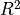is defined as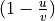, where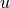is the residual sum of squares ((y_true - y_pred)** 2).sum() and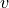is the total sum of squares ((y_true - y_true.mean()) ** 2).sum(). The best possible score is 1.0 and it can be negative (because the model can be arbitrarily worse). A constant model that always predicts the expected value of y, disregarding the input features, would get ascore of 0.0.

Parameters:
Xarray-like of shape (n_samples, n_features)

Test samples. For some estimators this may be a precomputed kernel matrix or a list of generic objects instead with shape (n_samples, n_samples_fitted), where n_samples_fitted is the number of samples used in the fitting for the estimator.

yarray-like of shape (n_samples,) or (n_samples, n_outputs)

True values for X.

sample_weightarray-like of shape (n_samples,), default=None

Sample weights.

Returns:
scorefloatof self.predict(X) w.r.t. y.

Notes

Thescore used when calling score on a regressor uses multioutput='uniform_average' from version 0.23 to keep consistent with default value of r2_score. This influences the score method of all the multioutput regressors (except for MultiOutputRegressor).

set_fit_request(*, sample_weight='$UNCHANGED$')#

Request metadata passed to the fit method.

Note that this method is only relevant if enable_metadata_routing=True (see sklearn.set_config). Please see User Guide on how the routing mechanism works.

The options for each parameter are:

• True: metadata is requested, and passed to fit if provided. The request is ignored if metadata is not provided.

• False: metadata is not requested and the meta-estimator will not pass it to fit.

• None: metadata is not requested, and the meta-estimator will raise an error if the user provides it.

• str: metadata should be passed to the meta-estimator with this given alias instead of the original name.

The default (sklearn.utils.metadata_routing.UNCHANGED) retains the existing request. This allows you to change the request for some parameters and not others.

New in version 1.3.

Note

This method is only relevant if this estimator is used as a sub-estimator of a meta-estimator, e.g. used inside a Pipeline. Otherwise it has no effect.

Parameters:
sample_weightstr, True, False, or None, default=sklearn.utils.metadata_routing.UNCHANGED

Metadata routing for sample_weight parameter in fit.

Returns:
selfobject

The updated object.

set_params(**params)#

Set the parameters of this estimator.

The method works on simple estimators as well as on nested objects (such as Pipeline). The latter have parameters of the form <component>__<parameter> so that it’s possible to update each component of a nested object.

Parameters:
**paramsdict

Estimator parameters.

Returns:
selfestimator instance

Estimator instance.

set_score_request(*, sample_weight='$UNCHANGED$')#

Request metadata passed to the score method.

Note that this method is only relevant if enable_metadata_routing=True (see sklearn.set_config). Please see User Guide on how the routing mechanism works.

The options for each parameter are:

• True: metadata is requested, and passed to score if provided. The request is ignored if metadata is not provided.

• False: metadata is not requested and the meta-estimator will not pass it to score.

• None: metadata is not requested, and the meta-estimator will raise an error if the user provides it.

• str: metadata should be passed to the meta-estimator with this given alias instead of the original name.

The default (sklearn.utils.metadata_routing.UNCHANGED) retains the existing request. This allows you to change the request for some parameters and not others.

New in version 1.3.

Note

This method is only relevant if this estimator is used as a sub-estimator of a meta-estimator, e.g. used inside a Pipeline. Otherwise it has no effect.

Parameters:
sample_weightstr, True, False, or None, default=sklearn.utils.metadata_routing.UNCHANGED

Metadata routing for sample_weight parameter in score.

Returns:
selfobject

The updated object.

## Examples using nilearn.decoding.SpaceNetRegressor#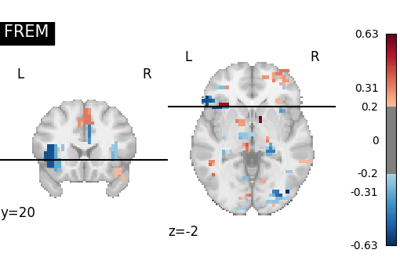FREM on Jimura et al “mixed gambles” dataset

FREM on Jimura et al "mixed gambles" dataset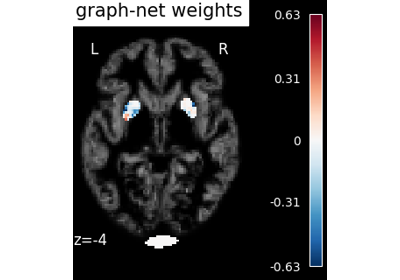Voxel-Based Morphometry on Oasis dataset with Space-Net prior

Voxel-Based Morphometry on Oasis dataset with Space-Net prior